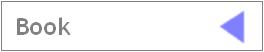PUBLICATIONS.Extending Intersection Homology Type Invariants to Non-Witt Spaces,                       Memoirs Amer. Math. Soc. 160 (2002), no. 760, pp. 1-83.Computing Twisted Signatures and L-Classes of Stratified Spaces                       (with S. Cappell and J. Shaneson),                       Math. Ann. 326 (2003), no. 3, pp. 589-623.Self-Dual Sheaves on the Reductive Borel-Serre Compactification                       of Hilbert Modular Surfaces.  (with Rajesh Kulkarni)                       Geom. Dedicata 105 (2004), pp. 121-141.Triangulations of 3-Dimensional Pseudomanifolds with an                       Application to State-Sum Invariants.  (with Greg Friedman)                       Algebr. Geom. Topology 4 (2004), pp. 521-542.Computing Twisted Signatures and L-Classes of Non-Witt Spaces.                        Proc. London Math. Soc. (3) 92 (2006), pp. 428-470.Generalized Arf Invariants in Algebraic L-Theory.  (with Andrew Ranicki),                        Adv. Math. 199 (2006), pp. 542-668.On Topological Invariants of Stratified Maps with non-Witt Target.                        Trans. Amer. Math. Soc. 358 (2006), no. 5, pp. 1921-1935.The L-Class of non-Witt Spaces.                        Annals of Math. 163 (2006), no. 3, pp. 743-766.Combinatorial Random Walks on 3-Manifolds.                        Experiment. Math. 15 (2006), no. 3, pp. 367-381.The Signature of Partially Defined Local Coefficient Systems,                       J. Knot Theory Ramifications 17 (2008), no. 12, pp. 1455 - 1481.Der topologische Raumbegriff in der Mathematik (doc),                       in Raumwissenschaften (Günzel, ed.), Suhrkamp Taschenbuch                       Wissenschaft 1891, Suhrkamp 2009.Rational Generalized Intersection Homology Theories,                       Homology, Homotopy Appl. 12 (2010), no. 1, pp. 157-185 (Journal Link, pdf).Singular Spaces and Generalized Poincaré Complexes,                       Electron. Res. Announc. Math. Sci. 16 (2009), pp. 63 - 73 (Journal Link, pdf).Intersection Spaces, Spatial Homology Truncation, and String Theory (pdf),                      Lecture Notes in Math. (vol. 1997), Springer Verlag, 2010.The Signature of Singular Spaces and its Refinements to Generalized Homology                       Theories (pdf), in Topology of Stratified Spaces (Friedman, Hunsicker, Libgober, Maxim, eds.),                       MSRI Publications (vol. 58), Cambridge University Press, New York, 2011.Knots, Singular Embeddings, and Monodromy (pdf) (with S. Cappell and J. Shaneson),                       in The Mathematics of Knots: Theory and Application (Banagl, Vogel, eds.), Contributions in Mathematical                       and Computational Sciences (vol. 1), Springer Verlag, 2011.The Mathematics of Knots: Theory and Application (with D. Vogel, eds.), Contributions in Mathematical                       and Computational Sciences (vol. 1), Springer Verlag, 2011.First Cases of Intersection Spaces in Stratification Depth 2 (pdf), J. of Singularities 5 (2012), pp. 57 - 84.Intersection Spaces and Hypersurface Singularities (pdf) (with L. Maxim), J. of Singularities 5 (2012), pp. 48 - 56.Deformation of Singularities and the Homology of Intersection Spaces (pdf) (with L. Maxim),                         J. Topol. Anal. 4 (2012), no. 4, pp. 413 - 448.The Tensor Product of Function Semimodules (pdf),                        Algebra Universalis 70 (2013), no. 3, pp. 213 - 226.Isometric Group Actions and the Cohomology of Flat Fiber Bundles (pdf),                        Groups Geom. Dyn. 7 (2013), no. 2, pp. 293 - 321.Intersection Spaces, Perverse Sheaves and Type IIB String Theory (pdf) (with N. Budur and L. Maxim),                        Adv. Theor. Math. Phys. 18 (2014), no. 2, pp. 363 - 399.Positive Topological Quantum Field Theories (pdf),                        Quantum Topology 6 (2015), no. 4, pp. 609 - 706.Refined Intersection Homology on non-Witt Spaces (pdf) (with P. Albin, E. Leichtnam, R. Mazzeo, P. Piazza),                       J. Topol. Anal. 7 (2015), no. 1, pp. 105 - 133.High-Dimensional Topological Field Theory, Positivity, and Exotic Smooth Spheres (pdf),                      technical report, University Heidelberg (2016).Foliated Stratified Spaces and a De Rham Complex Describing Intersection Space Cohomology (pdf),                       J. Differential Geometry 104 (2016), no. 1, pp. 1 - 58.Intersection Spaces, Equivariant Moore Approximation and the Signature (pdf),                       (with B. Chriestenson), J. of Singularities 16 (2017), pp. 141 - 179.Topological and Hodge L-Classes of Singular Covering Spaces and                      Varieties with Trivial Canonical Class (pdf), Geometriae Dedicata 199 (2019), pp. 189 - 224.The L-Homology Fundamental Class for IP-Spaces and the Stratified Novikov Conjecture (pdf)                       (with G. Laures and J. McClure), Selecta Math. 25:7 (2019), pp. 1 - 104.Hodge Theory for Intersection Space Cohomology (pdf)                      (with E. Hunsicker), Geometry & Topology 23 (2019), pp. 2165 - 2225.Stratified Formal Deformations and Intersection Homology of Data Point Clouds (pdf)                      (with T. Mäder and F. Sadlo), arXiv:2005.11985, 2020.Gysin Restriction of Topological and Hodge-Theoretic Characteristic Classes for Singular Spaces (pdf)                      New York J. of Math. 26 (2020), pp. 1273 - 1337.Bundle Transfer of L-Homology Orientation Classes for Singular Spaces (pdf)                      preprint (2022), arXiv:2210.05432The Uniqueness Theorem for Gysin Coherent Characteristic Classes of Singular Spaces (pdf)                      (with D. Wrazidlo), preprint (2022), arXiv:2210.13009Transfer and the Spectrum-Level Siegel-Sullivan KO-Orientation for Singular Spaces (pdf)                      preprint (2023), arXiv:2305.06045Home.